# GaussSiedel Method Major All Engineering Majors Authors Autar

• Slides: 35
Download presentation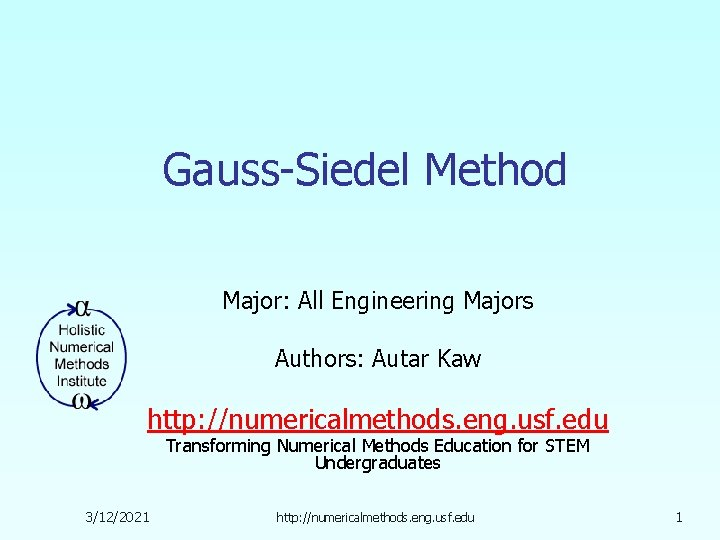Gauss-Siedel Method Major: All Engineering Majors Authors: Autar Kaw http: //numericalmethods. eng. usf. edu Transforming Numerical Methods Education for STEM Undergraduates 3/12/2021 http: //numericalmethods. eng. usf. edu 1Gauss-Seidel Method http: //numericalmethods. eng. usf. edu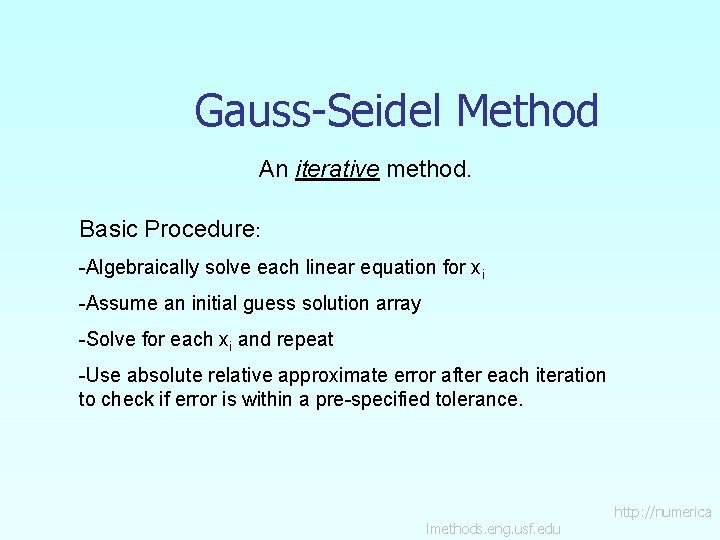Gauss-Seidel Method An iterative method. Basic Procedure: -Algebraically solve each linear equation for xi -Assume an initial guess solution array -Solve for each xi and repeat -Use absolute relative approximate error after each iteration to check if error is within a pre-specified tolerance. lmethods. eng. usf. edu http: //numericaGauss-Seidel Method Why? The Gauss-Seidel Method allows the user to control round-off error. Elimination methods such as Gaussian Elimination and LU Decomposition are prone to round-off error. Also: If the physics of the problem are understood, a close initial guess can be made, decreasing the number of iterations needed. lmethods. eng. usf. edu http: //numericaGauss-Seidel Method Algorithm A set of n equations and n unknowns: If: the diagonal elements are non-zero . . . Rewrite each equation solving for the corresponding unknown ex: First equation, solve for x 1 Second equation, solve for x 2 lmethods. eng. usf. edu http: //numericaGauss-Seidel Method Algorithm Rewriting each equation From Equation 1 From equation 2 From equation n-1 From equation n lmethods. eng. usf. edu http: //numerica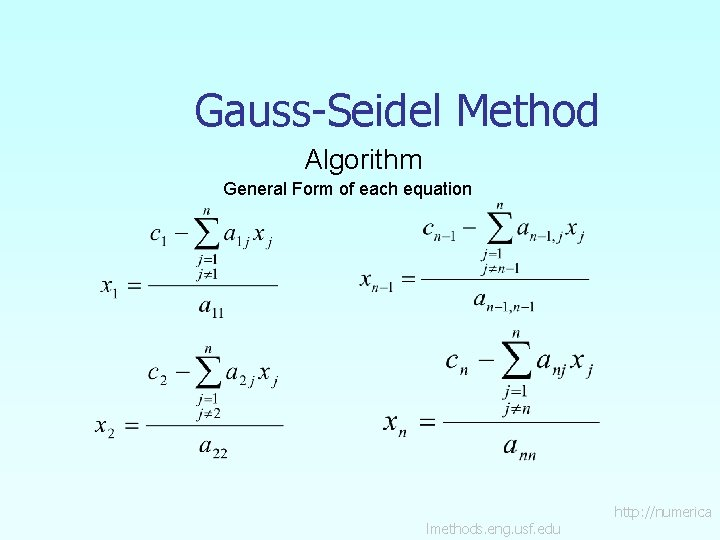Gauss-Seidel Method Algorithm General Form of each equation lmethods. eng. usf. edu http: //numericaGauss-Seidel Method Algorithm General Form for any row ‘i’ How or where can this equation be used? lmethods. eng. usf. edu http: //numericaGauss-Seidel Method Solve for the unknowns Assume an initial guess for [X] Use rewritten equations to solve for each value of xi. Important: Remember to use the most recent value of xi. Which means to apply values calculated to the calculations remaining in the current iteration. lmethods. eng. usf. edu http: //numericaGauss-Seidel Method Calculate the Absolute Relative Approximate Error So when has the answer been found? The iterations are stopped when the absolute relative approximate error is less than a prespecified tolerance for all unknowns. lmethods. eng. usf. edu http: //numerica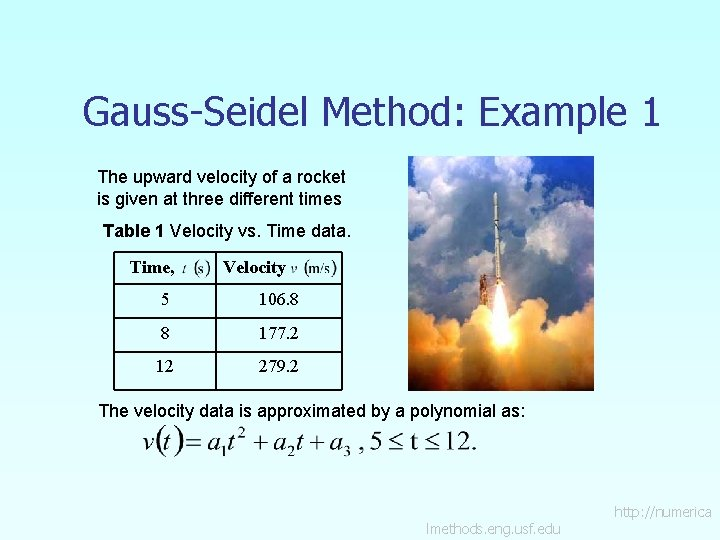Gauss-Seidel Method: Example 1 The upward velocity of a rocket is given at three different times Table 1 Velocity vs. Time data. Time, Velocity 5 106. 8 8 177. 2 12 279. 2 The velocity data is approximated by a polynomial as: lmethods. eng. usf. edu http: //numerica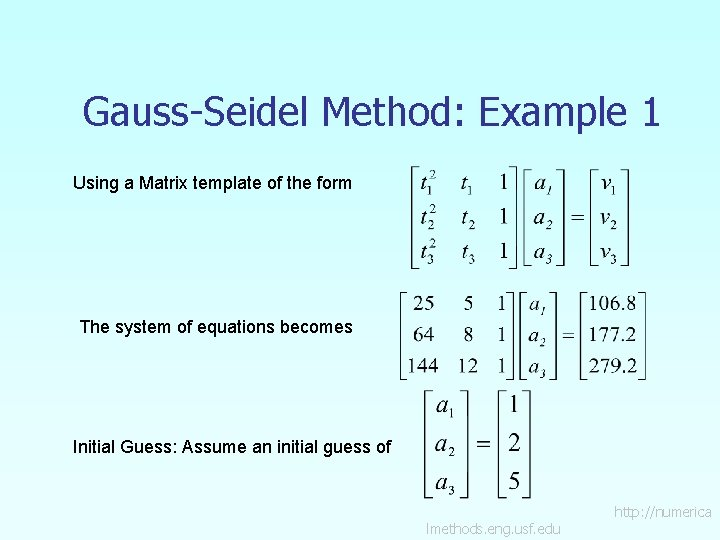Gauss-Seidel Method: Example 1 Using a Matrix template of the form The system of equations becomes Initial Guess: Assume an initial guess of lmethods. eng. usf. edu http: //numericaGauss-Seidel Method: Example 1 Rewriting each equation lmethods. eng. usf. edu http: //numericaGauss-Seidel Method: Example 1 Applying the initial guess and solving for ai Initial Guess When solving for a 2, how many of the initial guess values were used? lmethods. eng. usf. edu http: //numerica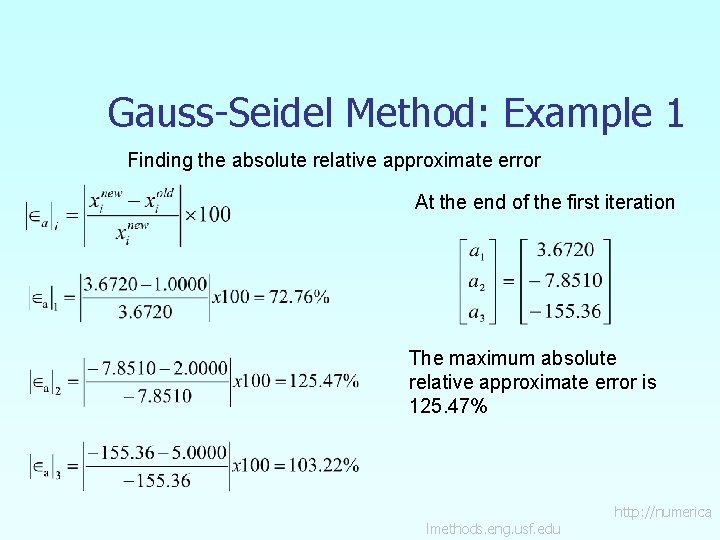Gauss-Seidel Method: Example 1 Finding the absolute relative approximate error At the end of the first iteration The maximum absolute relative approximate error is 125. 47% lmethods. eng. usf. edu http: //numerica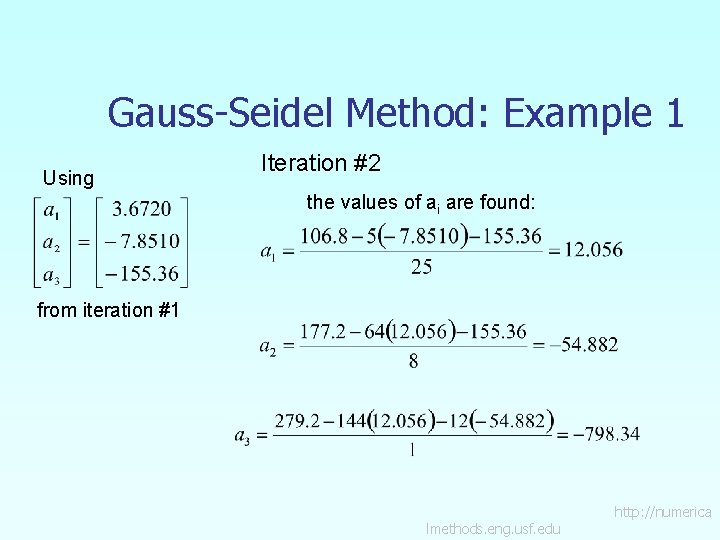Gauss-Seidel Method: Example 1 Using Iteration #2 the values of ai are found: from iteration #1 lmethods. eng. usf. edu http: //numerica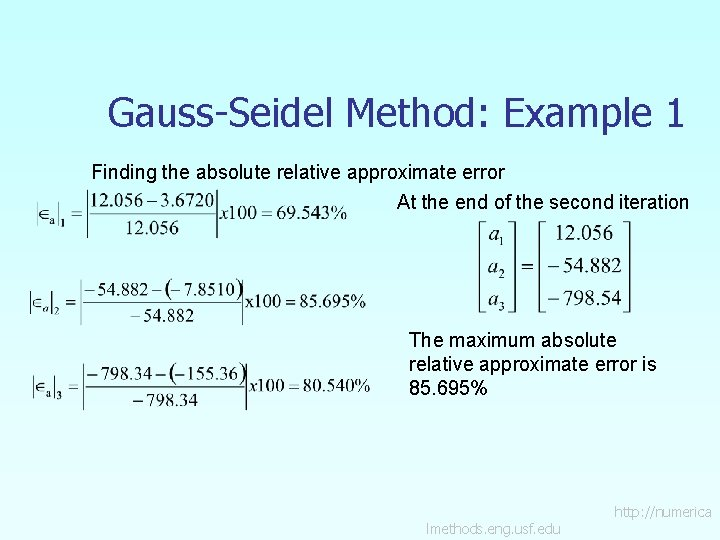Gauss-Seidel Method: Example 1 Finding the absolute relative approximate error At the end of the second iteration The maximum absolute relative approximate error is 85. 695% lmethods. eng. usf. edu http: //numerica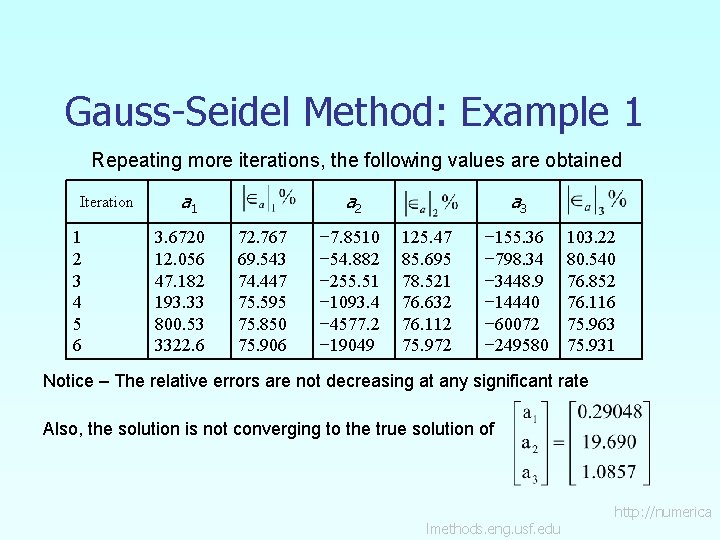Gauss-Seidel Method: Example 1 Repeating more iterations, the following values are obtained Iteration 1 2 3 4 5 6 a 1 3. 6720 12. 056 47. 182 193. 33 800. 53 3322. 6 a 2 72. 767 69. 543 74. 447 75. 595 75. 850 75. 906 − 7. 8510 − 54. 882 − 255. 51 − 1093. 4 − 4577. 2 − 19049 a 3 125. 47 85. 695 78. 521 76. 632 76. 112 75. 972 − 155. 36 − 798. 34 − 3448. 9 − 14440 − 60072 − 249580 103. 22 80. 540 76. 852 76. 116 75. 963 75. 931 Notice – The relative errors are not decreasing at any significant rate Also, the solution is not converging to the true solution of lmethods. eng. usf. edu http: //numericaGauss-Seidel Method: Pitfall What went wrong? Even though done correctly, the answer is not converging to the correct answer This example illustrates a pitfall of the Gauss-Siedel method: not all systems of equations will converge. Is there a fix? One class of system of equations always converges: One with a diagonally dominant coefficient matrix. Diagonally dominant: [A] in [A] [X] = [C] is diagonally dominant if: for all ‘i’ and for at least one ‘i’ lmethods. eng. usf. edu http: //numericaGauss-Seidel Method: Pitfall Diagonally dominant: The coefficient on the diagonal must be at least equal to the sum of the other coefficients in that row and at least one row with a diagonal coefficient greater than the sum of the other coefficients in that row. Which coefficient matrix is diagonally dominant? Most physical systems do result in simultaneous linear equations that have diagonally dominant coefficient matrices. lmethods. eng. usf. edu http: //numerica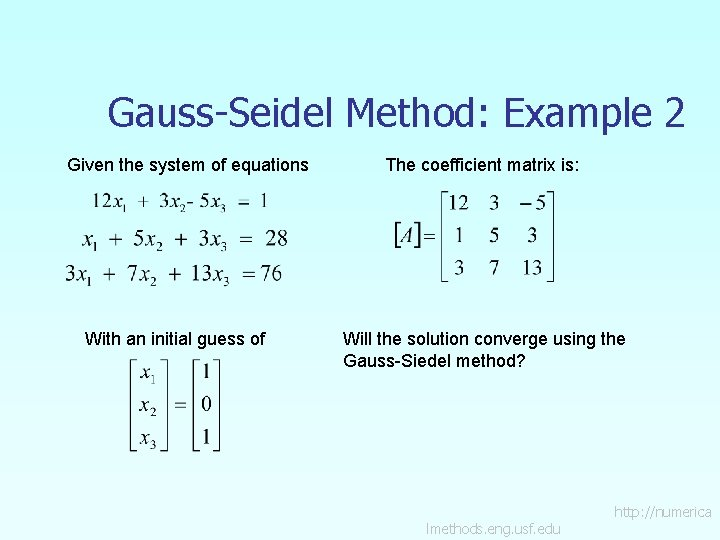Gauss-Seidel Method: Example 2 Given the system of equations With an initial guess of The coefficient matrix is: Will the solution converge using the Gauss-Siedel method? lmethods. eng. usf. edu http: //numerica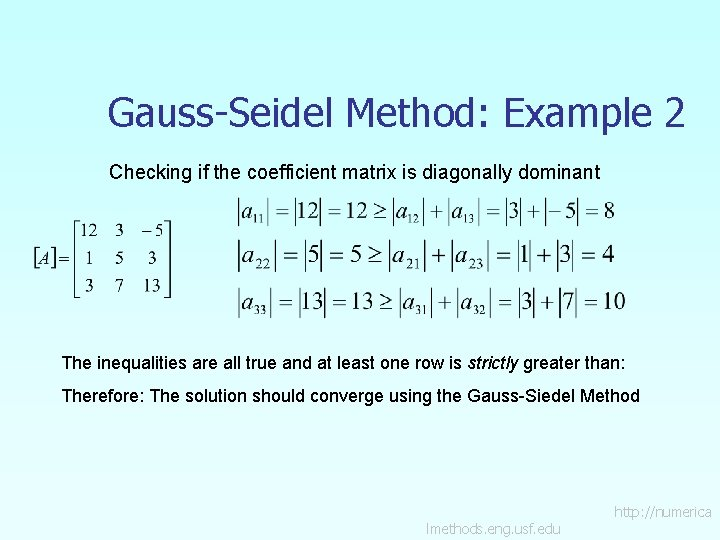Gauss-Seidel Method: Example 2 Checking if the coefficient matrix is diagonally dominant The inequalities are all true and at least one row is strictly greater than: Therefore: The solution should converge using the Gauss-Siedel Method lmethods. eng. usf. edu http: //numerica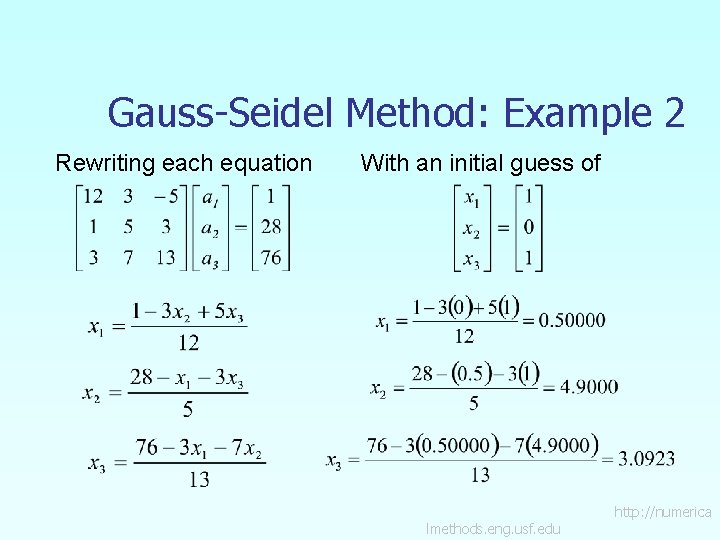Gauss-Seidel Method: Example 2 Rewriting each equation With an initial guess of lmethods. eng. usf. edu http: //numerica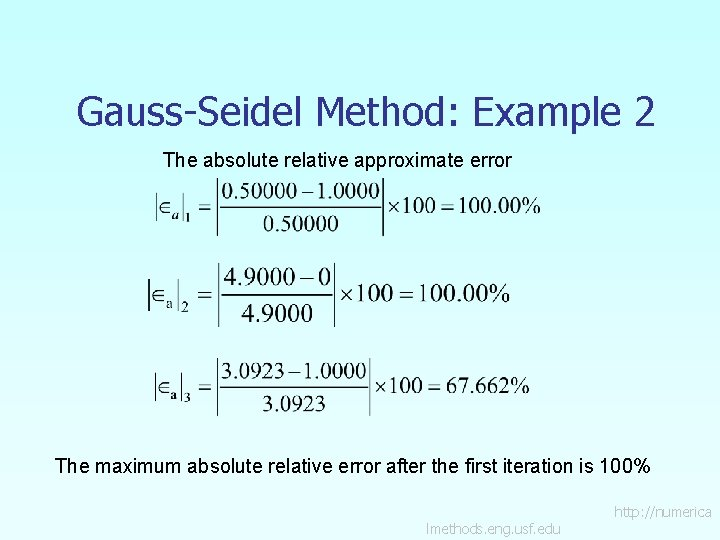Gauss-Seidel Method: Example 2 The absolute relative approximate error The maximum absolute relative error after the first iteration is 100% lmethods. eng. usf. edu http: //numerica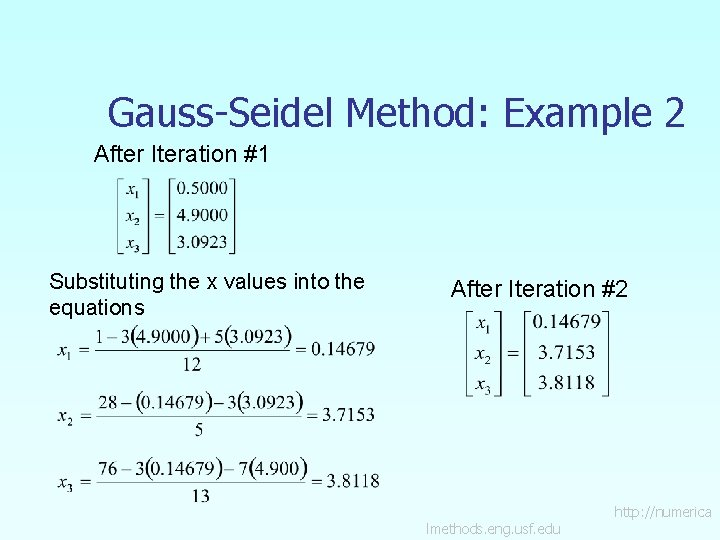Gauss-Seidel Method: Example 2 After Iteration #1 Substituting the x values into the equations After Iteration #2 lmethods. eng. usf. edu http: //numericaGauss-Seidel Method: Example 2 Iteration #2 absolute relative approximate error The maximum absolute relative error after the first iteration is 240. 61% This is much larger than the maximum absolute relative error obtained in iteration #1. Is this a problem? lmethods. eng. usf. edu http: //numericaGauss-Seidel Method: Example 2 Repeating more iterations, the following values are obtained Iteration a 1 1 2 3 4 5 6 0. 50000 0. 14679 0. 74275 0. 94675 0. 99177 0. 99919 The solution obtained a 2 100. 00 240. 61 80. 236 21. 546 4. 5391 0. 74307 4. 9000 3. 7153 3. 1644 3. 0281 3. 0034 3. 0001 a 3 100. 00 31. 889 17. 408 4. 4996 0. 82499 0. 10856 3. 0923 3. 8118 3. 9708 3. 9971 4. 0001 67. 662 18. 876 4. 0042 0. 65772 0. 074383 0. 00101 is close to the exact solution of lmethods. eng. usf. edu . http: //numerica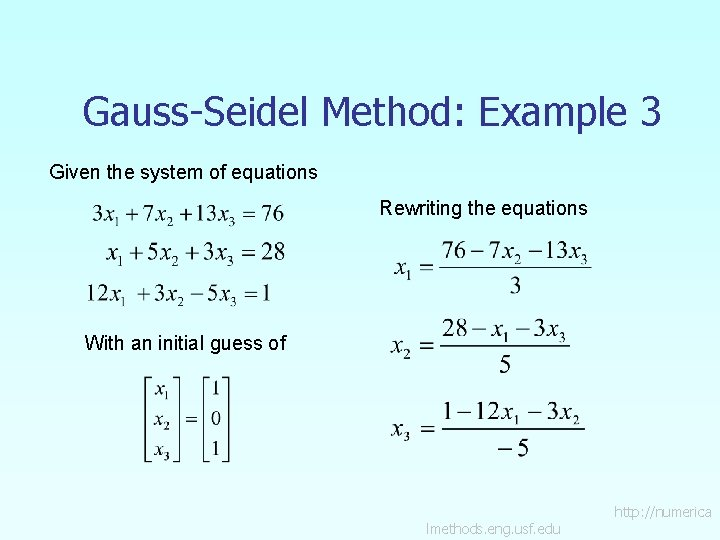Gauss-Seidel Method: Example 3 Given the system of equations Rewriting the equations With an initial guess of lmethods. eng. usf. edu http: //numerica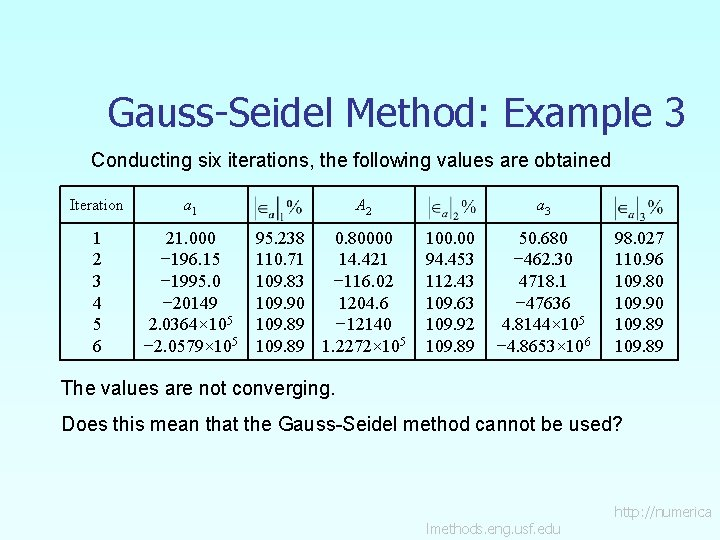Gauss-Seidel Method: Example 3 Conducting six iterations, the following values are obtained Iteration a 1 1 2 3 4 5 6 21. 000 − 196. 15 − 1995. 0 − 20149 2. 0364× 105 − 2. 0579× 105 A 2 95. 238 0. 80000 110. 71 14. 421 109. 83 − 116. 02 109. 90 1204. 6 109. 89 − 12140 109. 89 1. 2272× 105 a 3 100. 00 94. 453 112. 43 109. 63 109. 92 109. 89 50. 680 − 462. 30 4718. 1 − 47636 4. 8144× 105 − 4. 8653× 106 98. 027 110. 96 109. 80 109. 90 109. 89 The values are not converging. Does this mean that the Gauss-Seidel method cannot be used? lmethods. eng. usf. edu http: //numericaGauss-Seidel Method The Gauss-Seidel Method can still be used The coefficient matrix is not diagonally dominant But this is the same set of equations used in example #2, which did converge. If a system of linear equations is not diagonally dominant, check to see if rearranging the equations can form a diagonally dominant matrix. lmethods. eng. usf. edu http: //numerica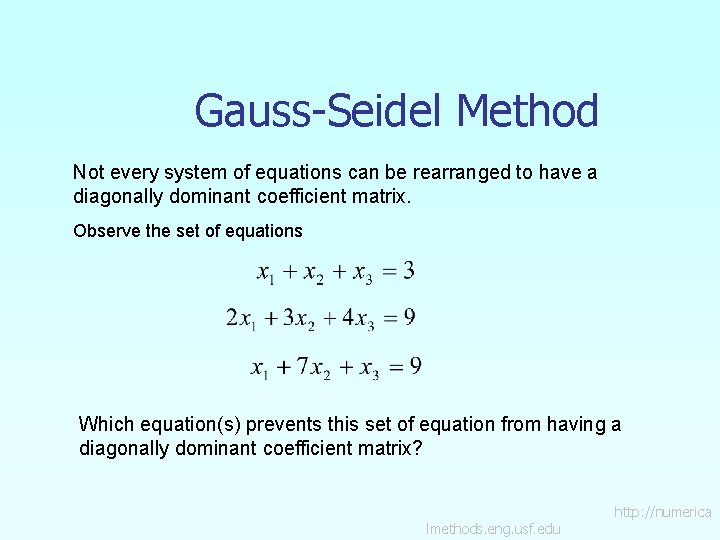Gauss-Seidel Method Not every system of equations can be rearranged to have a diagonally dominant coefficient matrix. Observe the set of equations Which equation(s) prevents this set of equation from having a diagonally dominant coefficient matrix? lmethods. eng. usf. edu http: //numerica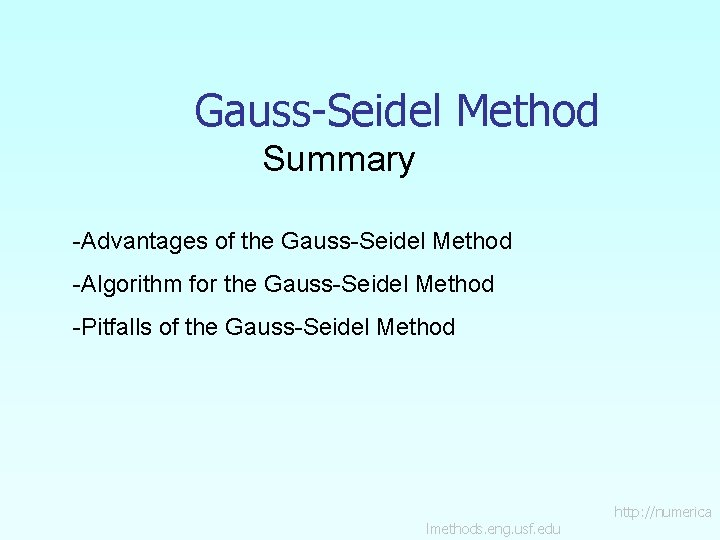Gauss-Seidel Method Summary -Advantages of the Gauss-Seidel Method -Algorithm for the Gauss-Seidel Method -Pitfalls of the Gauss-Seidel Method lmethods. eng. usf. edu http: //numerica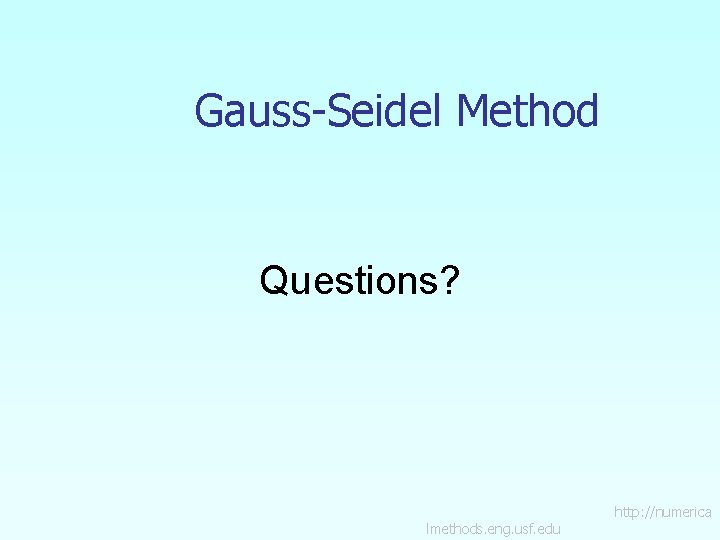Gauss-Seidel Method Questions? lmethods. eng. usf. edu http: //numericaAdditional Resources For all resources on this topic such as digital audiovisual lectures, primers, textbook chapters, multiple-choice tests, worksheets in MATLAB, MATHEMATICA, Math. Cad and MAPLE, blogs, related physical problems, please visit http: //numericalmethods. eng. usf. edu/topics/gauss_seid el. html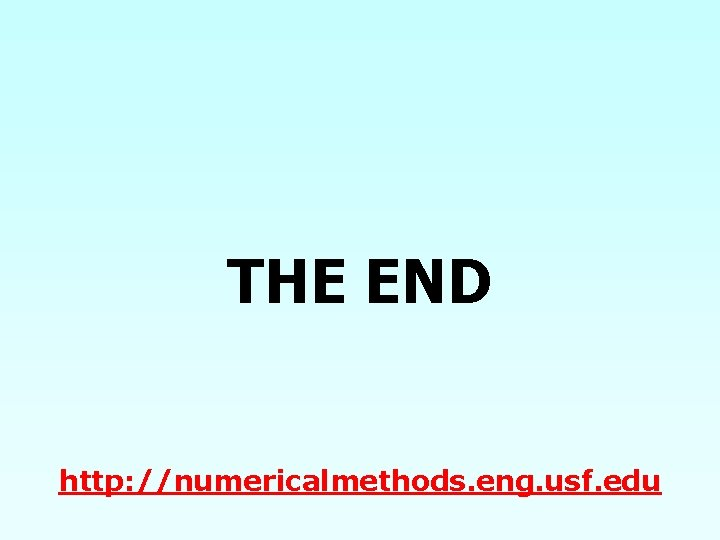THE END http: //numericalmethods. eng. usf. edu